# Voltage Measurement 1 Voltmeter A voltmeter is an

• Slides: 44
Download presentation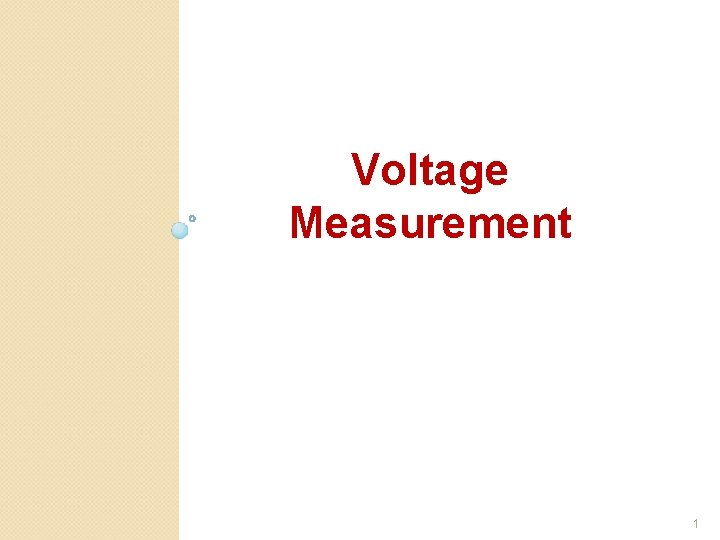Voltage Measurement 1Voltmeter A voltmeter is an instrument used for measuring the potential difference between two points in an electric circuit. 2A voltmeter is placed in parallel with a circuit element to measure the voltage drop across it and must be designed to draw very little current from the circuit so that it does not appreciably change the circuit it is measuring. To accomplish this, a large resistor is placed in series with the galvanometer. Its value is chosen so that the design voltage placed across the meter will cause the meter to deflect to its full-scale reading. A galvanometer full-scale current is very small: on the order of milliamperes. 3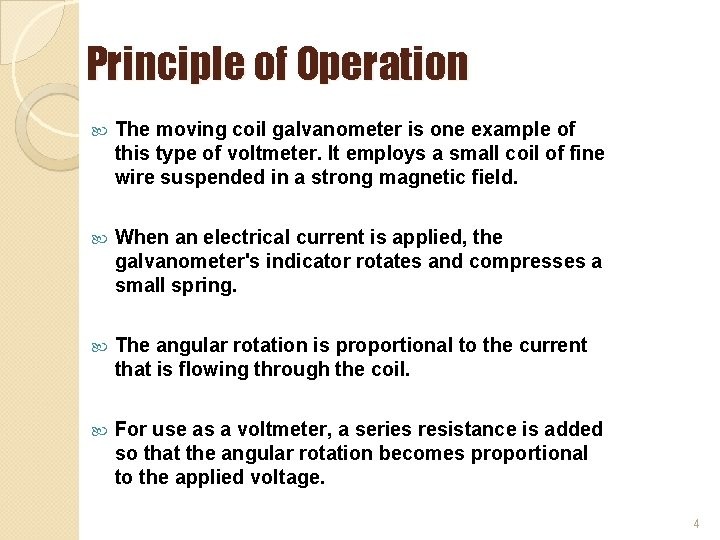Principle of Operation The moving coil galvanometer is one example of this type of voltmeter. It employs a small coil of fine wire suspended in a strong magnetic field. When an electrical current is applied, the galvanometer's indicator rotates and compresses a small spring. The angular rotation is proportional to the current that is flowing through the coil. For use as a voltmeter, a series resistance is added so that the angular rotation becomes proportional to the applied voltage. 4D’Ársonval Meter Movement In A DC Voltmeter The basic d’Ársonval meter movement can be converted to a dc voltmeter by connecting a multiplier Rs in series with the meter movement The purpose of the multiplier: ◦ to extend the voltage range of the meter ◦ to limit current through the d’Arsonval meter movement to a maximum full-scale deflection current. 5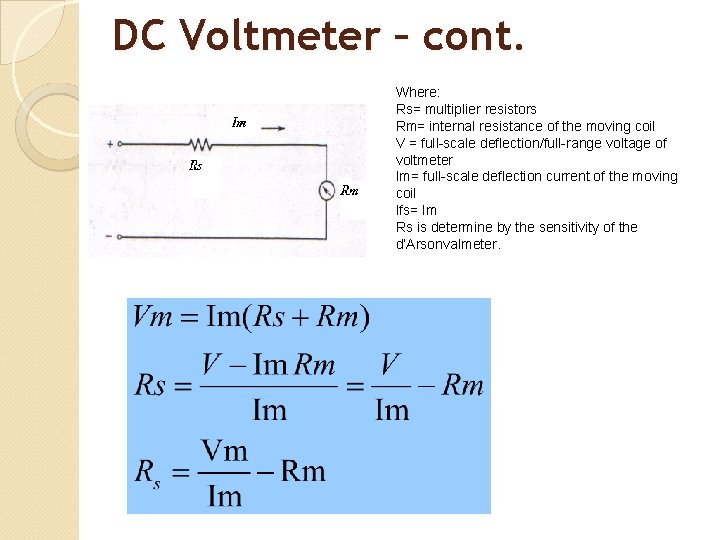DC Voltmeter – cont. Where: Rs= multiplier resistors Rm= internal resistance of the moving coil V = full-scale deflection/full-range voltage of voltmeter Im= full-scale deflection current of the moving coil Ifs= Im Rs is determine by the sensitivity of the d’Arsonvalmeter.To find the value of the multiplier resistor, first determine the sensitivity, S, of the meter movement. 7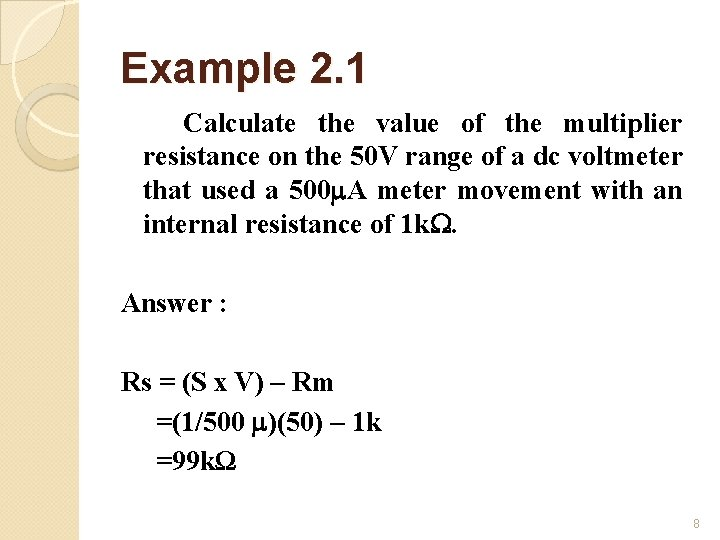Example 2. 1 Calculate the value of the multiplier resistance on the 50 V range of a dc voltmeter that used a 500 A meter movement with an internal resistance of 1 k. Answer : Rs = (S x V) – Rm =(1/500 )(50) – 1 k =99 kΩ 8Meter movements D’Arsonval meter movement having a full-scale deflection rating of 1 m. A and a coil resistance of 500 Ω, using Ohm’s Law (E=IR). Determine how much voltage will drive this meter movement directly to full scale: E = I R E = (1 m. A)(500 Ω) E = 0. 5 voltsThe series resistor is called a “multiplier” resistor because it multiplies the working range of the meter movement as it proportionately divides the measured voltage across it.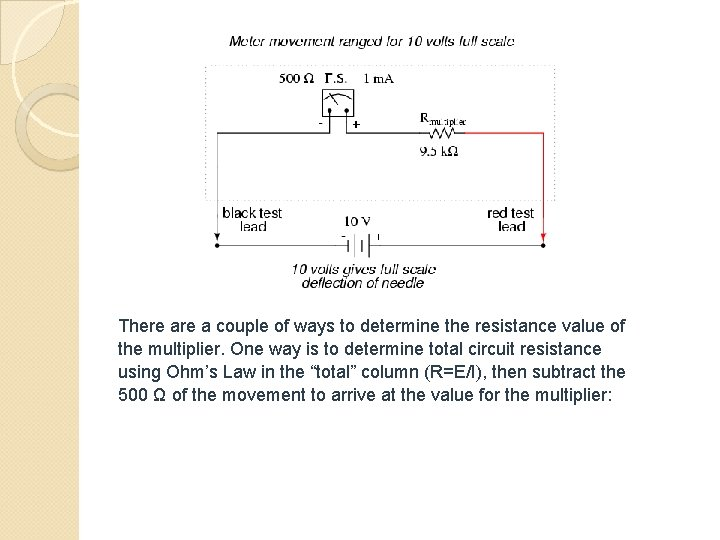There a couple of ways to determine the resistance value of the multiplier. One way is to determine total circuit resistance using Ohm’s Law in the “total” column (R=E/I), then subtract the 500 Ω of the movement to arrive at the value for the multiplier: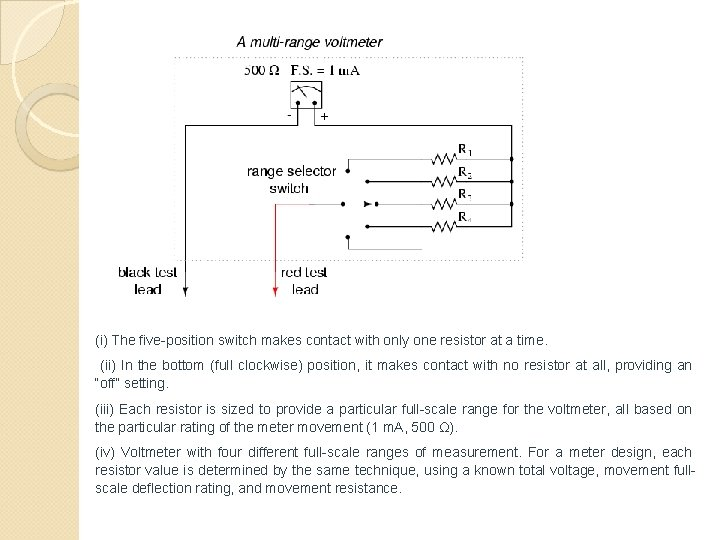(i) The five-position switch makes contact with only one resistor at a time. (ii) In the bottom (full clockwise) position, it makes contact with no resistor at all, providing an “off” setting. (iii) Each resistor is sized to provide a particular full-scale range for the voltmeter, all based on the particular rating of the meter movement (1 m. A, 500 Ω). (iv) Voltmeter with four different full-scale ranges of measurement. For a meter design, each resistor value is determined by the same technique, using a known total voltage, movement fullscale deflection rating, and movement resistance.(i) Voltmeter with ranges of 1 volt, 10 volts, 100 volts, and 1000 volts. (ii) To determine R 1, R 2, R 3 & R 4: using formula Ohm’s Law in (R=E/I), then subtract the 500 Ω of the movement to arrive at the value for the multiplier. (iii) Rtotal = R 1+R 2+R 3+R 4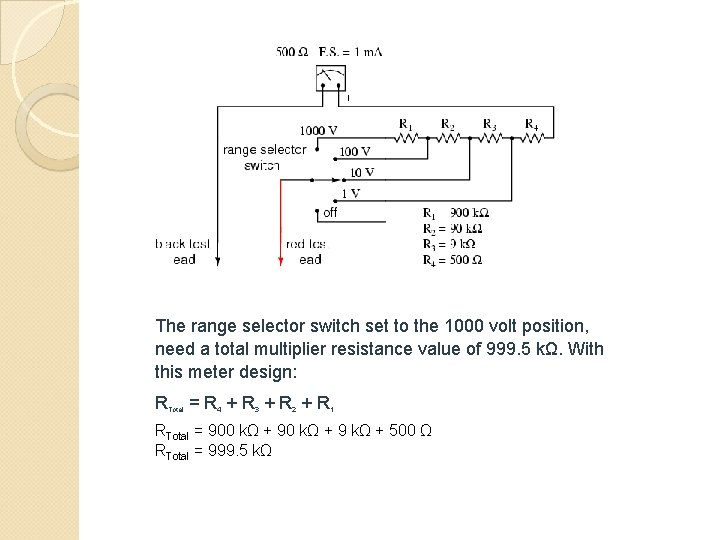The range selector switch set to the 1000 volt position, need a total multiplier resistance value of 999. 5 kΩ. With this meter design: RTotal = R 4 + R 3 + R 2 + R 1 RTotal = 900 kΩ + 9 kΩ + 500 Ω RTotal = 999. 5 kΩ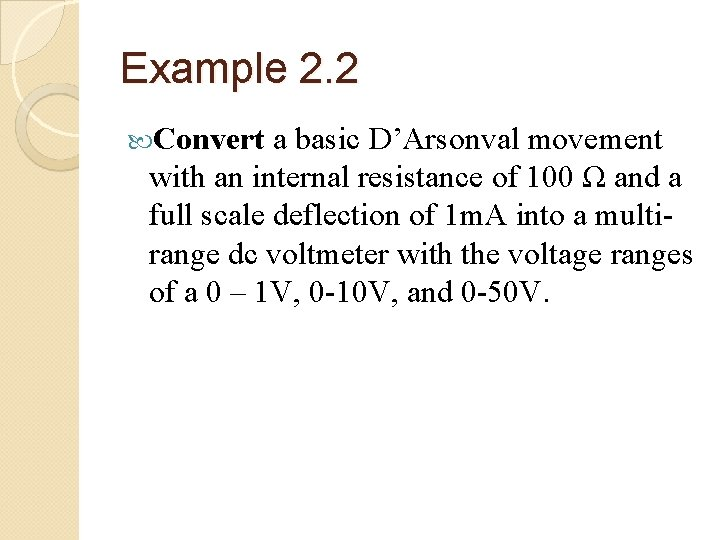Example 2. 2 Convert a basic D’Arsonval movement with an internal resistance of 100 Ω and a full scale deflection of 1 m. A into a multirange dc voltmeter with the voltage ranges of a 0 – 1 V, 0 -10 V, and 0 -50 V.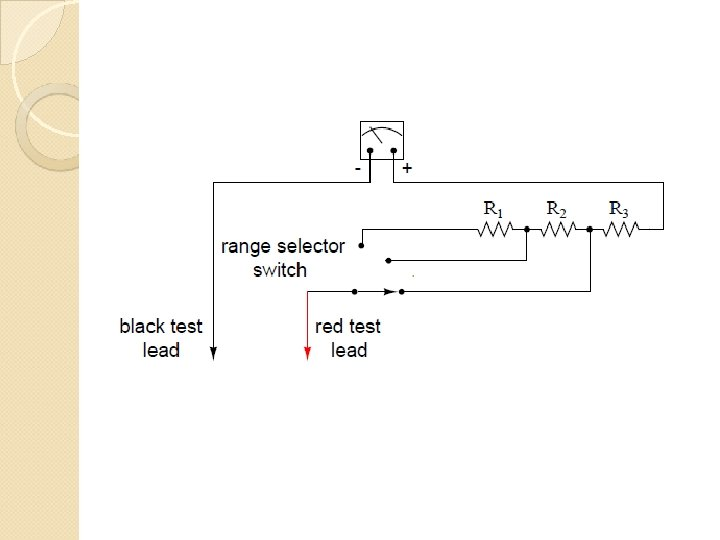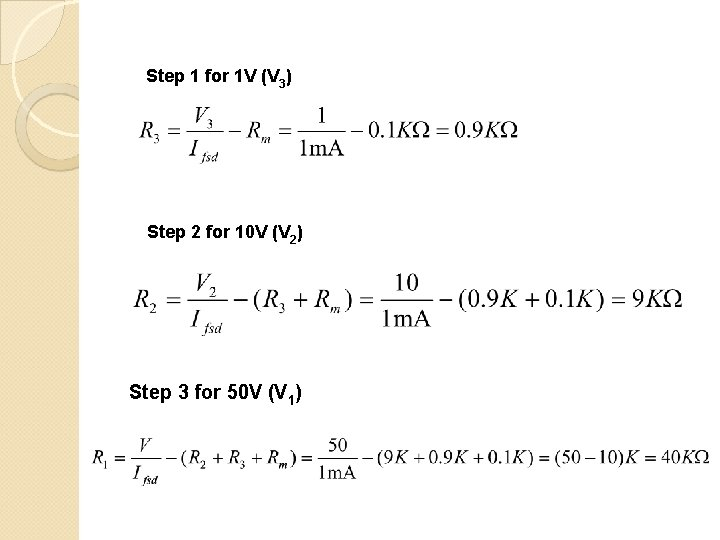Step 1 for 1 V (V 3) Step 2 for 10 V (V 2) Step 3 for 50 V (V 1)Exercises 1) A D’Arsonval movement with a full scale deflection of 50µA and having an internal resistance of 500 Ω is to be converted into a multirange voltmeter. Determine the value of multiplier required for 0 -20 V, 0 – 50 V and 0100 V using individual multipliers for each range. Calculate the values of the individual resistor. 1) Convert a basic D’Arsonval movement with a full scale deflection of 10 m. A and having an internal resistance of 100 Ω into a multirange voltmeter with ranges from 0 -5 V, 0 – 50 V and 0 - 100 V 18Voltmeter Loading Effects Voltmeter is used to measure the voltage across a circuit component, the voltmeter circuit is parallel with the circuit component. Since the parallel combination of two resistors is less than either resistor alone, the resistance seen by the source is less with the voltmeter connected than without. Therefore, the voltage across the component is less whenever the voltmeter is connected. The decrease in voltage may be negligible or it may be appreciable, depending on the sensitivity of the voltmeter being used. This effect is called voltmeter loading. The resulting error is called a loading error. 19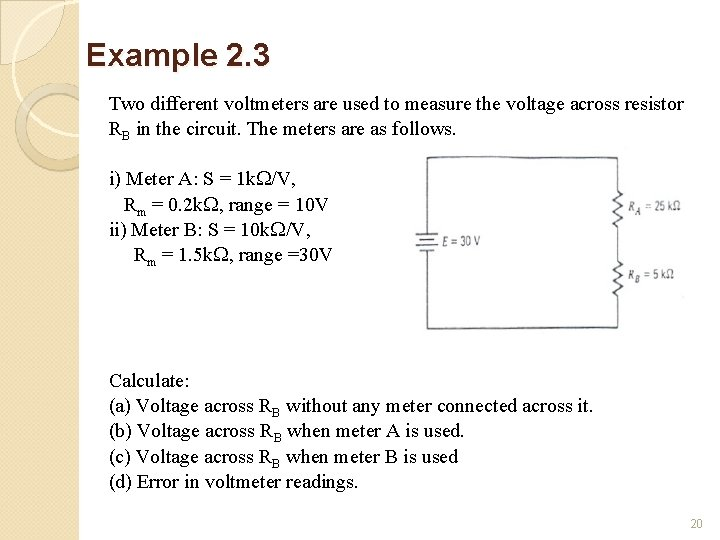Example 2. 3 Two different voltmeters are used to measure the voltage across resistor RB in the circuit. The meters are as follows. i) Meter A: S = 1 k /V, Rm = 0. 2 k , range = 10 V ii) Meter B: S = 10 k /V, Rm = 1. 5 k , range =30 V Calculate: (a) Voltage across RB without any meter connected across it. (b) Voltage across RB when meter A is used. (c) Voltage across RB when meter B is used (d) Error in voltmeter readings. 20Solution (a) The voltage across resistor RB without either meter connected is found Using the voltage divider equation: 21(b) starting with meter A, the total resistance it presents to the circuit is The parallel combination of RB and meter A is Therefore, the voltage reading obtained with meter A, determined by the voltage divider equation, is 22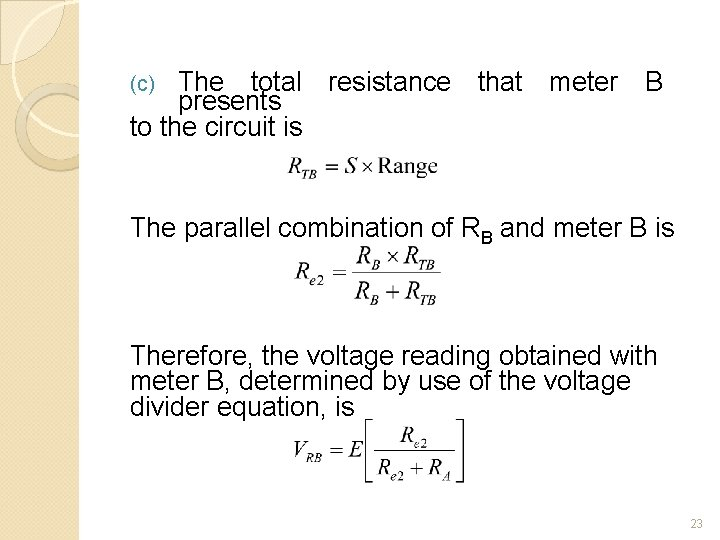The total resistance that meter B presents to the circuit is (c) The parallel combination of RB and meter B is Therefore, the voltage reading obtained with meter B, determined by use of the voltage divider equation, is 23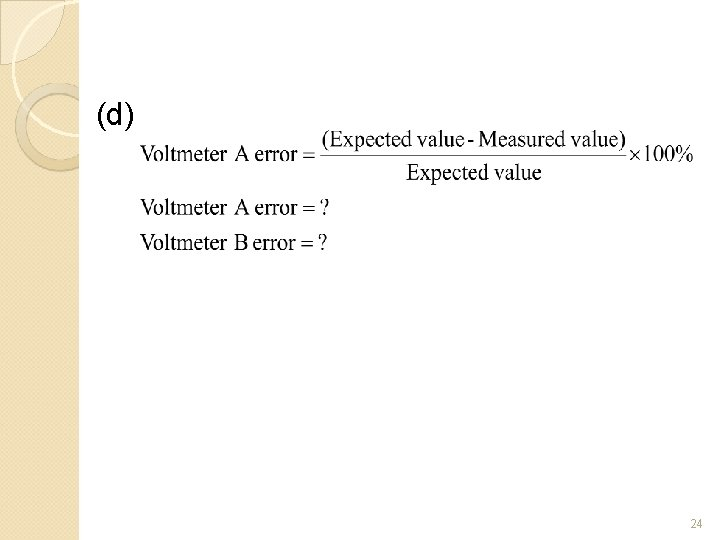(d) 24Five principal meter movements used in ac instrument 1. Electrodynamometer 2. Iron Vane 3. Electrostatic 4. Thermocouple 5. D’Arsonval with rectifier 25Application of meter movements Meter Movement DC Use Applications Electrodynamometer YES Standards meter, wattmeter, frequency meter “Indicator” applications such as in automobiles Iron Vane YES “Indicator” applications such as in automobiles Electrostatic YES Measurement of high voltage when very little current can be supplied by the circuit being measured Thermocouple YES Measurement of radio frequency ac signal D’Arsonval YES with rectifier Most widely used meter movement for measuring direct current or voltage and resistance 26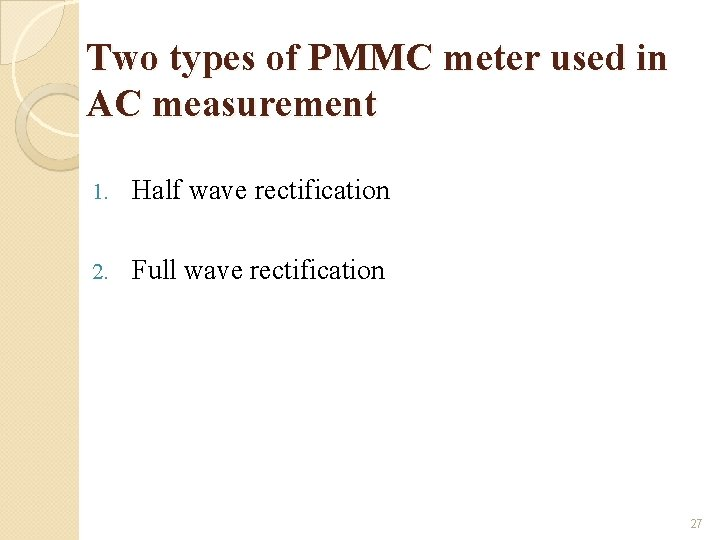Two types of PMMC meter used in AC measurement 1. Half wave rectification 2. Full wave rectification 27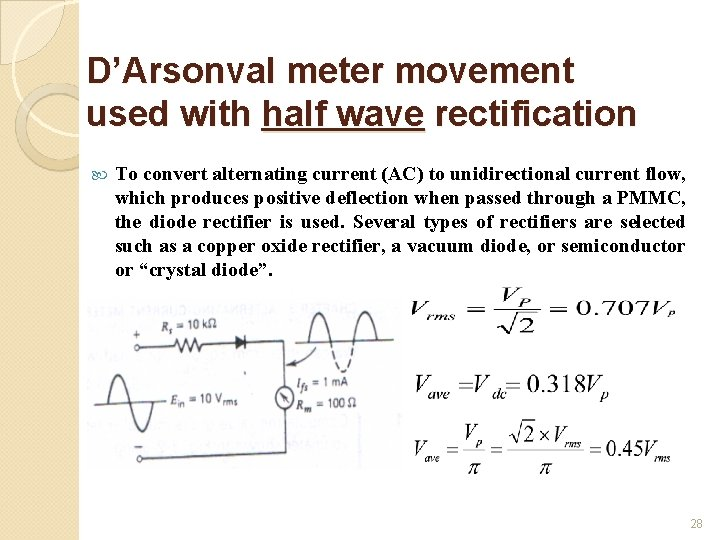D’Arsonval meter movement used with half wave rectification To convert alternating current (AC) to unidirectional current flow, which produces positive deflection when passed through a PMMC, the diode rectifier is used. Several types of rectifiers are selected such as a copper oxide rectifier, a vacuum diode, or semiconductor or “crystal diode”. 28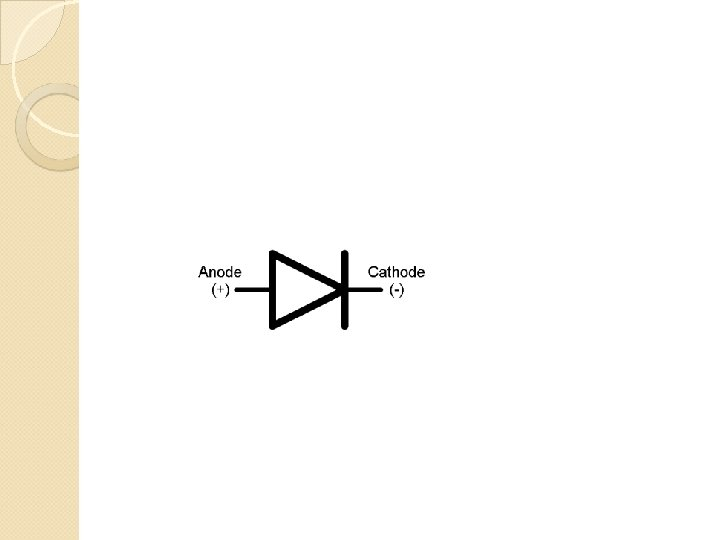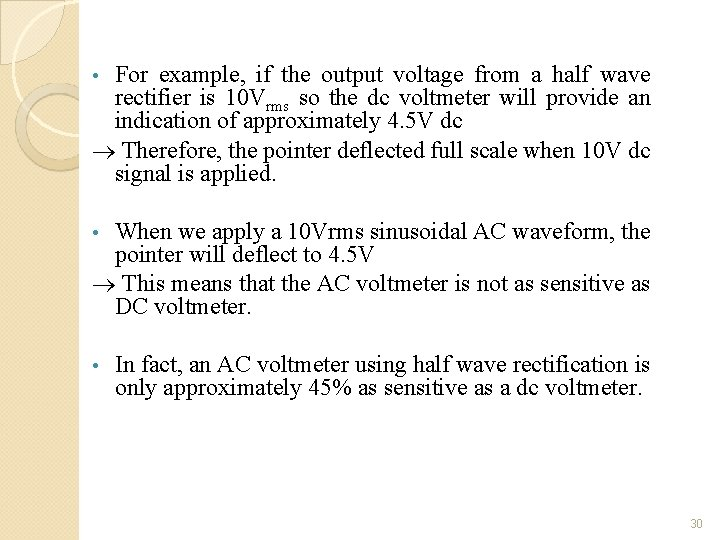For example, if the output voltage from a half wave rectifier is 10 Vrms so the dc voltmeter will provide an indication of approximately 4. 5 V dc Therefore, the pointer deflected full scale when 10 V dc signal is applied. • When we apply a 10 Vrms sinusoidal AC waveform, the pointer will deflect to 4. 5 V This means that the AC voltmeter is not as sensitive as DC voltmeter. • • In fact, an AC voltmeter using half wave rectification is only approximately 45% as sensitive as a dc voltmeter. 30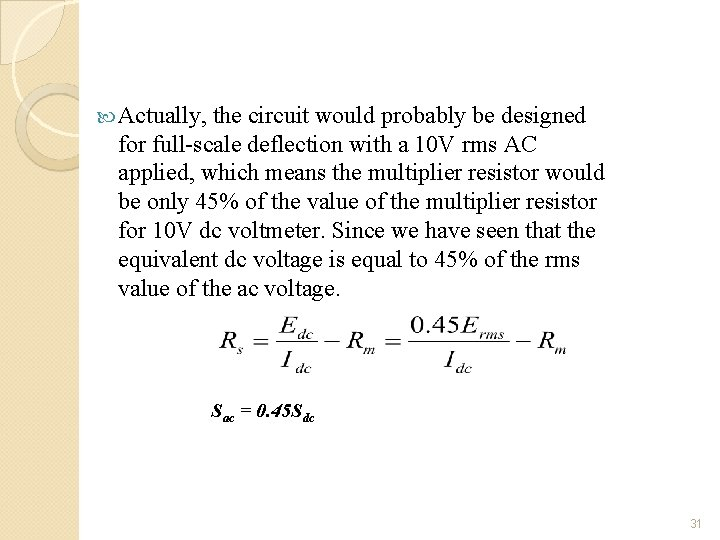Actually, the circuit would probably be designed for full-scale deflection with a 10 V rms AC applied, which means the multiplier resistor would be only 45% of the value of the multiplier resistor for 10 V dc voltmeter. Since we have seen that the equivalent dc voltage is equal to 45% of the rms value of the ac voltage. Sac = 0. 45 Sdc 31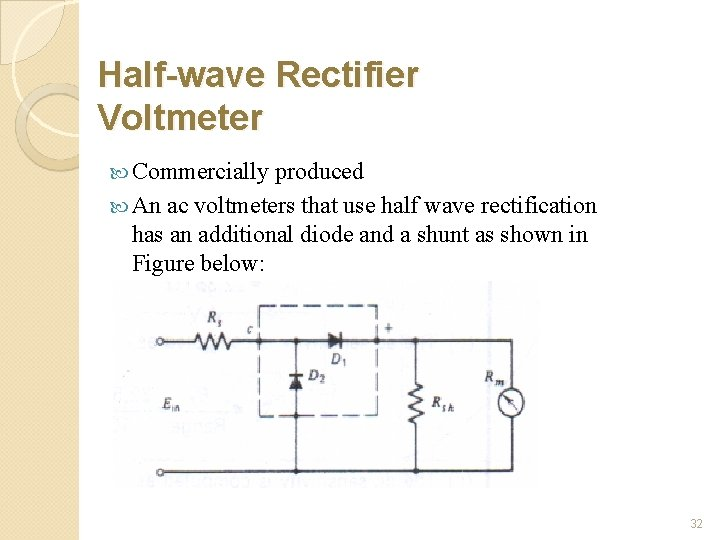Half-wave Rectifier Voltmeter Commercially produced An ac voltmeters that use half wave rectification has an additional diode and a shunt as shown in Figure below: 32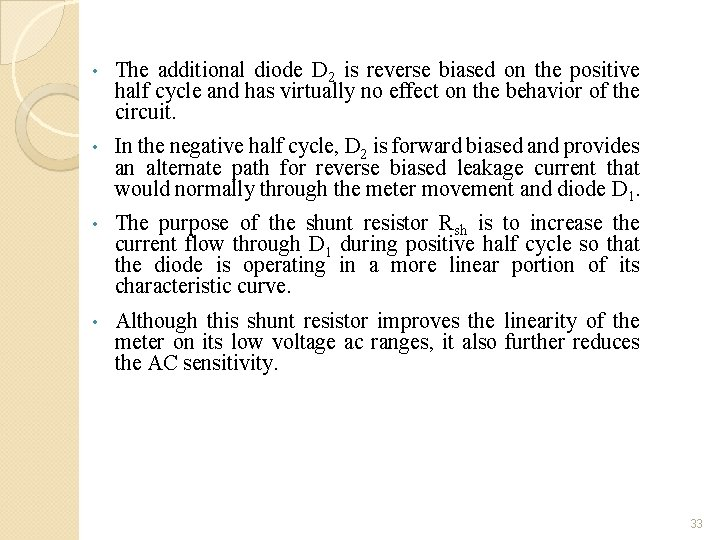• The additional diode D 2 is reverse biased on the positive half cycle and has virtually no effect on the behavior of the circuit. • In the negative half cycle, D 2 is forward biased and provides an alternate path for reverse biased leakage current that would normally through the meter movement and diode D 1. • The purpose of the shunt resistor Rsh is to increase the current flow through D 1 during positive half cycle so that the diode is operating in a more linear portion of its characteristic curve. • Although this shunt resistor improves the linearity of the meter on its low voltage ac ranges, it also further reduces the AC sensitivity. 33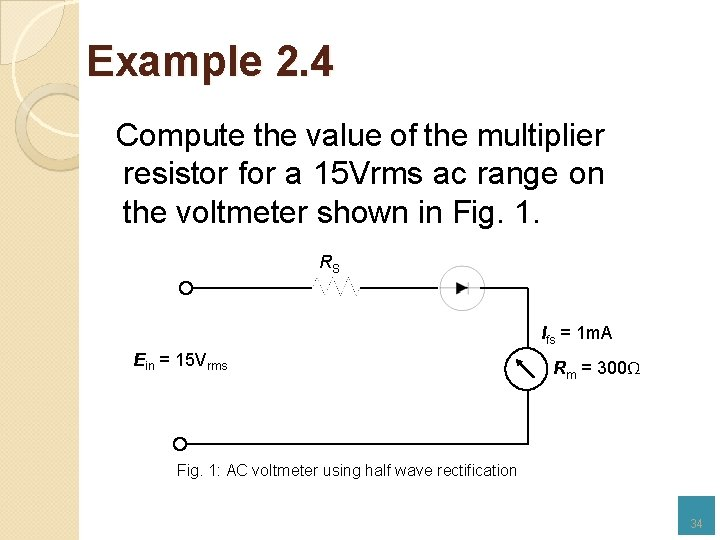Example 2. 4 Compute the value of the multiplier resistor for a 15 Vrms ac range on the voltmeter shown in Fig. 1. RS Ifs = 1 m. A Ein = 15 Vrms Rm = 300Ω Fig. 1: AC voltmeter using half wave rectification 34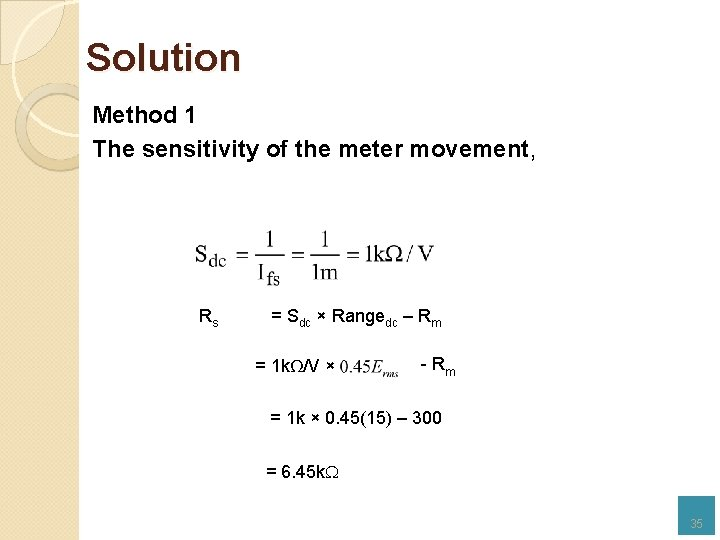Solution Method 1 The sensitivity of the meter movement, Rs = Sdc × Rangedc – Rm = 1 kΩ/V × - Rm = 1 k × 0. 45(15) – 300 = 6. 45 k 35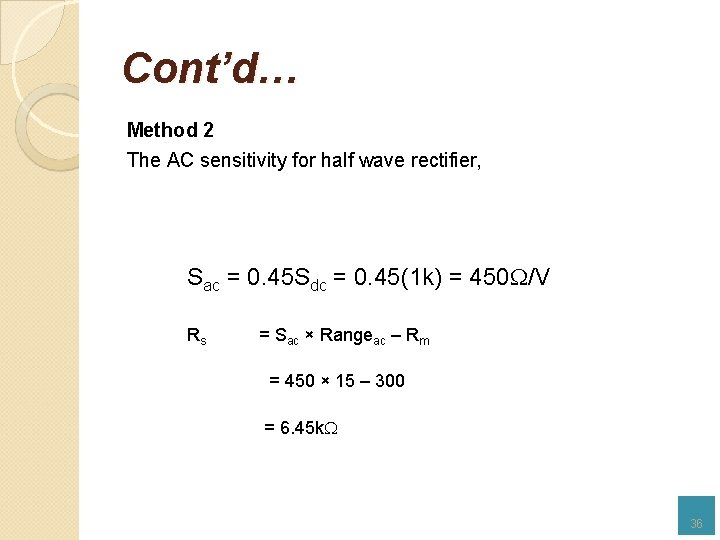Cont’d… Method 2 The AC sensitivity for half wave rectifier, Sac = 0. 45 Sdc = 0. 45(1 k) = 450 /V Rs = Sac × Rangeac – Rm = 450 × 15 – 300 = 6. 45 k 36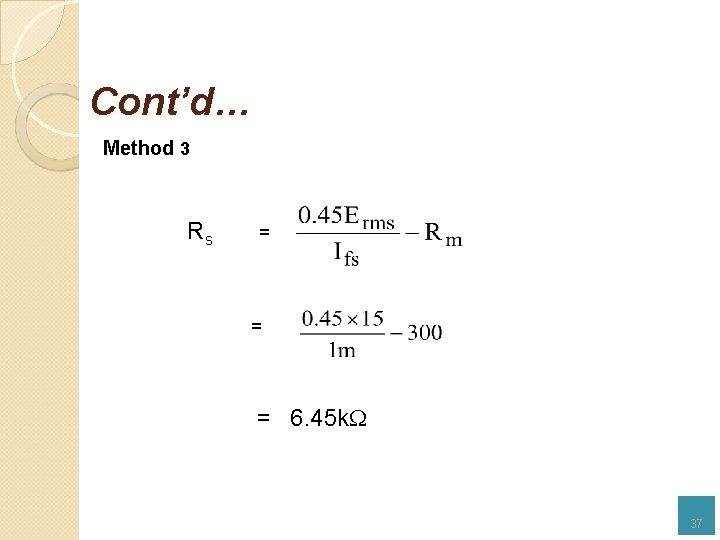Cont’d… Method 3 Rs = = = 6. 45 k 37D’Arsonval meter movement used with full wave rectification Fig. 2: Full bridge rectifier used in an ac voltmeter circuit During the positive half cycle, currents flows through diode D 2, through the meter movement from positive to negative, and through diode D 3. The polarities in circles on the transformer secondary are for the positive half cycle. Since current flows through the meter movement on both half cycles, can expect the deflection of the pointer to be greater than with the half wave cycle, which allows current to flow only on every other half cycle; if the deflection remains the same, the instrument using full wave rectification will have a greater sensitivity. 38Consider the circuit shown in Fig. 1 -2: AC voltmeter using full wave rectification 39Cont’d… When the 10 Vrms of AC signal is applied to the circuit above, where the peak value of the AC input signal is And the average full wave output signal is Therefore, we can see that a 10 Vrms voltage is equivalent to 9 Vdc for full-scale deflection. 40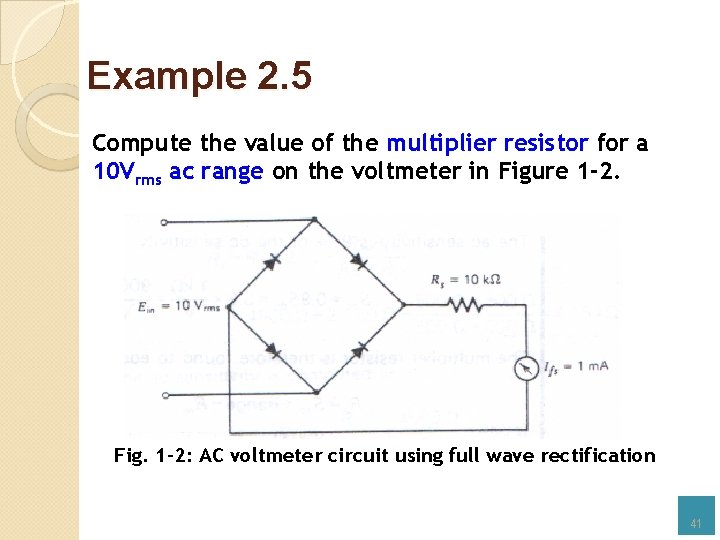Example 2. 5 Compute the value of the multiplier resistor for a 10 Vrms ac range on the voltmeter in Figure 1 -2. Fig. 1 -2: AC voltmeter circuit using full wave rectification 41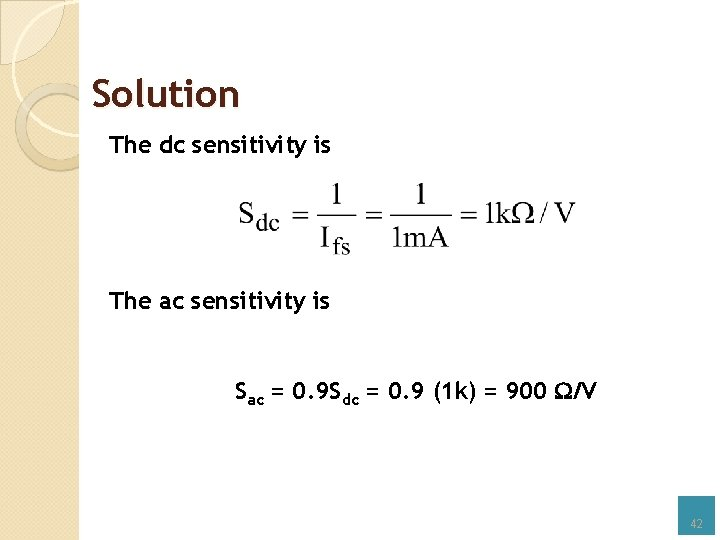Solution The dc sensitivity is The ac sensitivity is Sac = 0. 9 Sdc = 0. 9 (1 k) = 900 /V 42Cont’d… Therefore the multiplier resistor is Rs = Sac x Range – Rm = 900 x 10 Vrms – 500 = 8. 5 k 43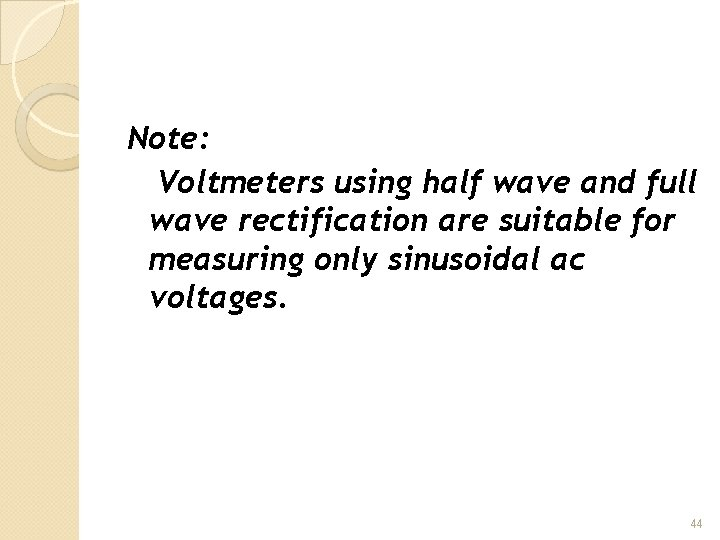Note: Voltmeters using half wave and full wave rectification are suitable for measuring only sinusoidal ac voltages. 44